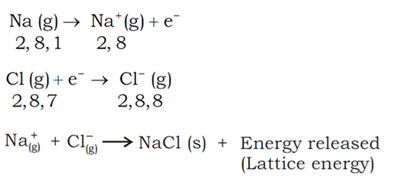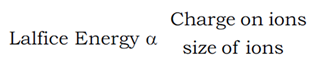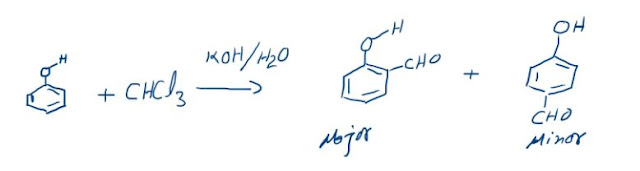## Ionic Bond

(a) Chemical bond formed between ions is called ionic bond.
(b) Ionic bonds are formed by loss and gain of e– between two atoms.
(c) The electrostatic force of attraction developed between the cation and anion is known as ionic bond. It is also called as electrovalent bond due to involvement of electrostatic forces of attraction.
(d) Electrovalent bond is not possible between similar atoms. This type of bonding requires two atoms of different nature. One atom should have the tendency to lose electron i.e. electropositive in nature and the other atom should have the tendency to accept electron.Conditions for forming ionic bond

1. Low ionisation energy of one atom.

2. High electron affinity of other atom.

3. High Lattice energy of compound formed.

Now for stable ionic bonding the total energy released should be more than the energy required.
(a) Ionization energy: I
n the formation of ionic bond a metal atom loses electron to form cation. This process required energy equal to the ionization energy. Lesser the value of ionization energy, greater is the tendency of the atom to form cation. For example, alkali metals form cations quite easily because of the low values of ionization energies.

(b) Electron affinity: Electron affinity is the energy released when gaseous atom accepts electron to form a negative ion. Thus, the value of electron affinity gives the tendency of an atom to form anion. Now greater the value of electron affinity more is the tendency of an atom to form anion. For example, halogens having highest electron affinities within their respective periods to form ionic compounds with metals very easily.
(c) Lattice energy: Once the gaseous ions are formed, the ions of opposite charges come close together and pack up three dimensionally in a definite geometric pattern to form ionic crystal.

Lattice energy

(a) The amount of energy released when free ions combine together to form one mole of a crystal is called lattice energy (v)
M+ (g) + X(g) à M+X(S)
(b) Higher the lattice energy greater will be the ease of formation of the ionic compound.

(c) Factors influencing magnitude of lattice energy are as follows:

(i) Size of ions: Smaller the size of the ions, lesser is the internuclear distance.
Consequently the interionic attraction will be high and lattice energy will also be large. e.g. NaCl > KCl

(ii) Charge on ions: Larger the magnitudes of charge on the ions, greater will be the attractive forces between the ions. Consequently the lattice energy will be high. e.g. L.E. of MgO > L.E of Na2O### The Reimer-Tiemann reaction: Reaction and its Mechanism

Reimer-Tiemann Reaction Phenols on reaction with chloroform in the presence of sodium hydroxide (or potassium hydroxide) solution give hydro...